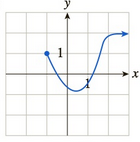Chapter 10.2, Problem 17EFinite Mathematics and Applied Cal...

7th Edition
Stefan Waner + 1 other
ISBN: 9781337274203

Solutions

Chapter
SectionFinite Mathematics and Applied Cal...

7th Edition
Stefan Waner + 1 other
ISBN: 9781337274203
Textbook Problem

In Exercises 17–24, the graph of a function f is given. Deter- mine whether, at the given point a, f is continuous, discontinuous, or singular. [HINT: See Quick Examples 5 and 6.]a = − 1

To determine

Whether the function f is continuous, discontinuous or singular at the point a=1. The graph of function f is as follows:Explanation

Given information:

The graph is as follows:

From the graph, it is seen that the limit does exist for each of the point in the domain as the graph of function f is break free and smooth in its entire domain.

To check the continuity at a=1, evaluate the left limit and right limit.

As x is approaching 1 from the right, it is noticed from the graph that y-coordinate approaches 1 this means limx1+f(x)=1.

As the left-limit does not exist because there is no point in the domain to the left of x=1.

From the above evaluation, it is concluded that the limit does exist for x=1 and equals to the right limit as the limit is estimated near the point x=1 and in the domain of f

Still sussing out bartleby?

Check out a sample textbook solution.

See a sample solution

The Solution to Your Study Problems

Bartleby provides explanations to thousands of textbook problems written by our experts, many with advanced degrees!

Get Started